# Help With Learning Algebra: Pass the parcel

March 11, 2014 by Mark MaclaineAfter Mark Maclaine’s first blog post explaining how to understand the basics of algebra, he now goes into more depth using his ‘pass the parcel’ method in order to supply another method to help with learning algebra.

As a kid I remember playing a game called “pass the parcel” which involved unwrapping a present layer by layer every time the music stopped. It’s one of those games kids play at parties, and can lead to tantrums…. But what has this got to do with algebra?

Imagine that the side of the equation containing the unknown (the letter) is a bit like that parcel. It has been wrapped in a set of layers one by one. The order that it was wrapped in can be determined by BIDMAS or BODMAS. See my blog post on how to understand BIDMAS here:

For example: 3m + 5

In this case, let’s say m = 10: the first thing we would do is multiply it by 3 (as multiplication comes before addition), and then we would add 5. Now if we were to try “unwrap” this in an equation, just like the parcel in the game, we would have to unwrap it in the reverse order: last layer first, and so on…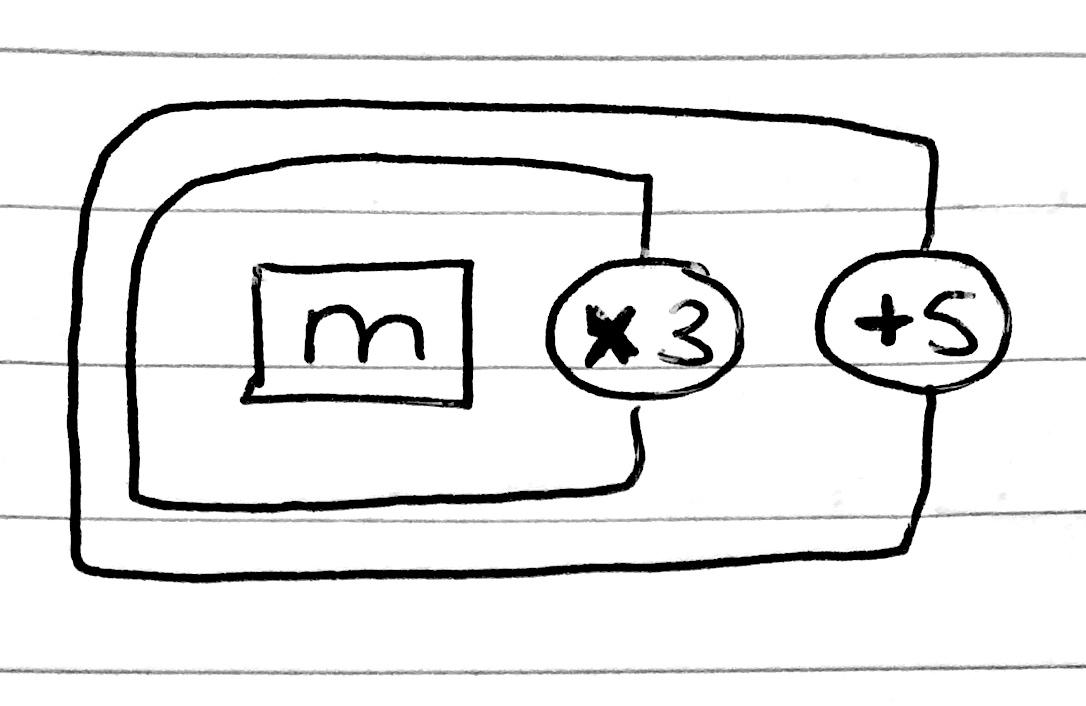As we can see the last layer to be wrapped was the “add 5”, so this is the first layer we take off. Only then can we remove the “multiply by 3”. If m was placed into the following equation, the first thing we could get rid of is the “add 5”. Remember, the opposite of adding 5 is subtracting it: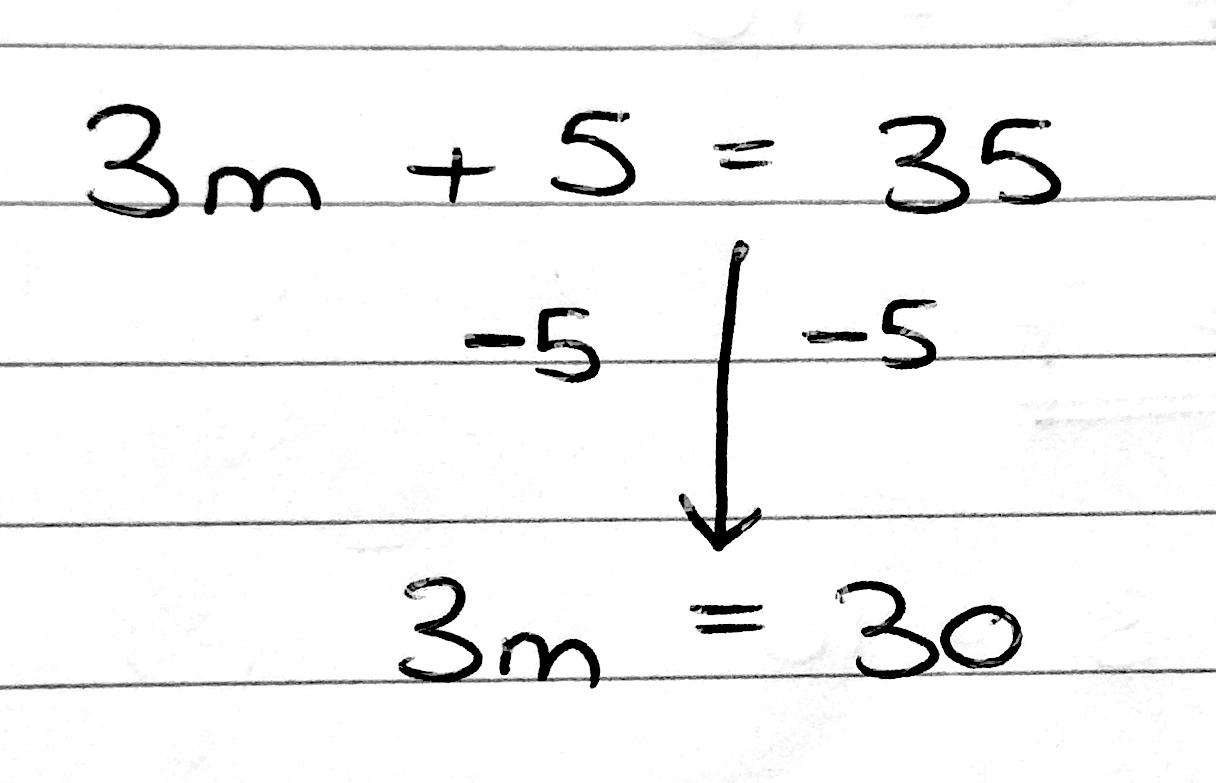From here we are now left with the final layer – this is the “multiply by 3”, so to get rid of that we do the opposite – we “divide by 3”.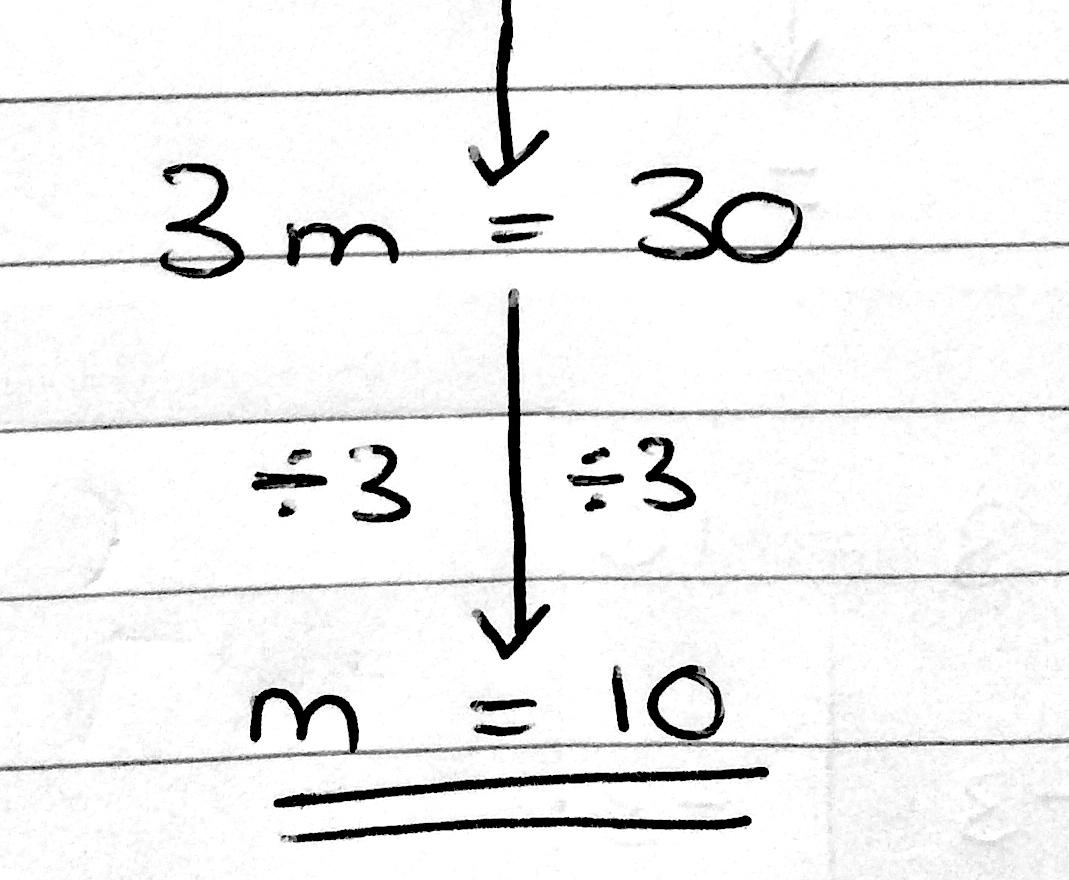Now let’s look at a slightly more complicated question.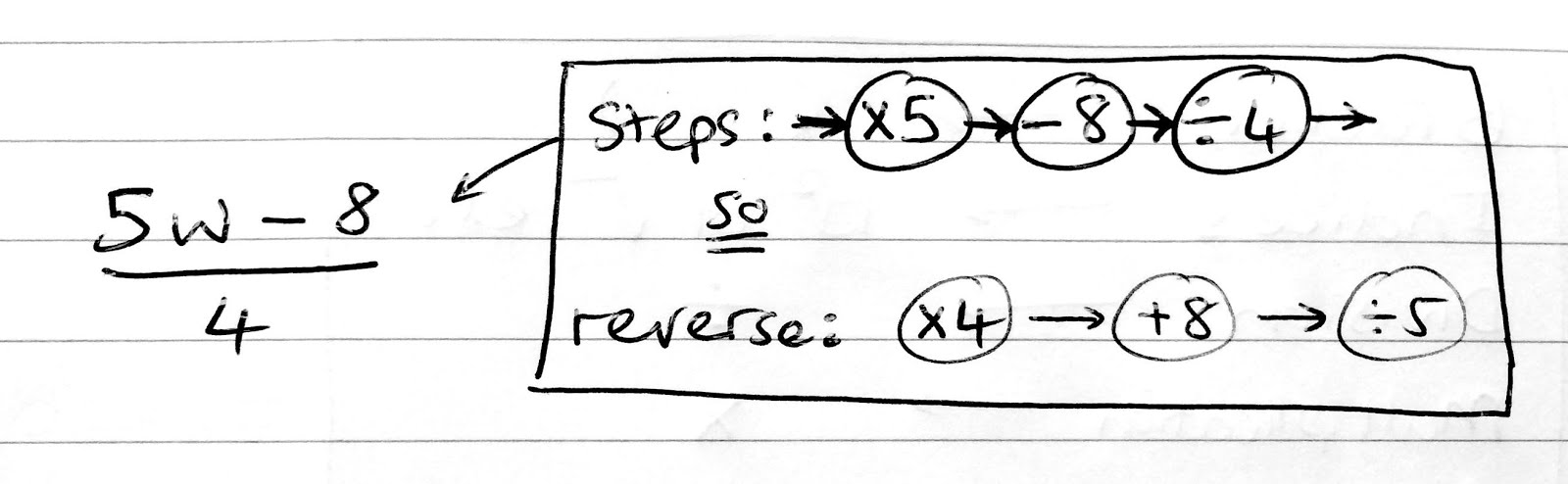In the case above, the w is first multiplied by 5, then you subtract 8 and only then divide everything by 4. This may be a little bit confusing; following the rules of BIDMAS, division normally comes first but because the whole sum  ‘5w-8’ is divided by four (as if there were brackets/parentheses around it) this is done last.

Remember: the first thing to unwrap is the last thing that was wrapped: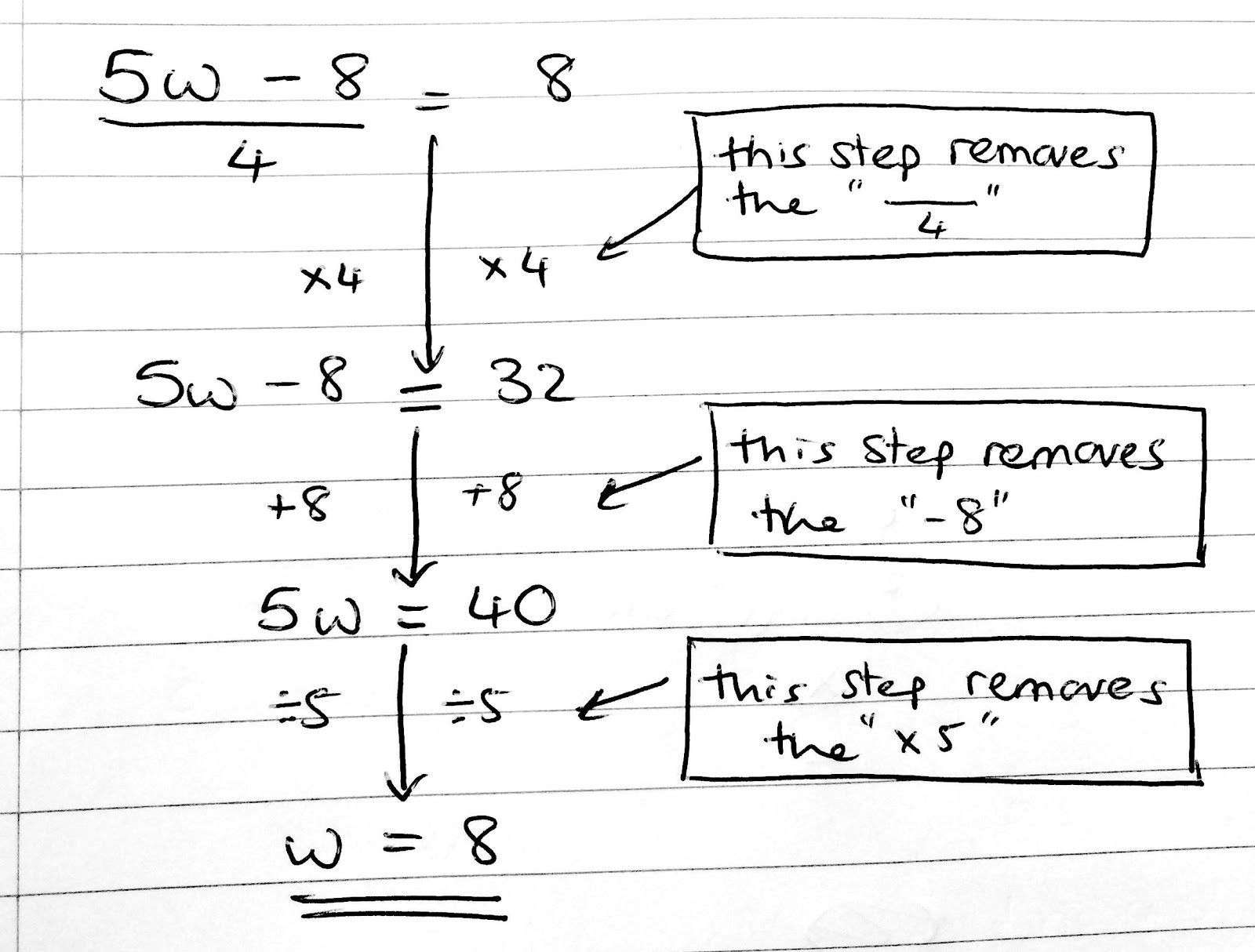This method takes a little time to get your head around, but once you have it, even hard questions (like the dreaded square roots!) become a whole lot easier.

Let’s look at this final example:How has this been wrapped? First ‘m’ was multiplied by 3, then 4 was added 4 and only then was the square root of the whole thing put in.

Like the previous example: normally when following the rules of BIDMAS, indices (squares, square roots and other powers/roots) come before multiplication and addition. However, because the whole sum ‘3m+4’ is contained inside the root sign (as if contained within brackets/parenthses) it is done first, and only then is it square-rooted. Now to unwrap the equation and solve it, we must do everything in reverse order.

Note: the opposite of square-root is to square, and vice-versa. So…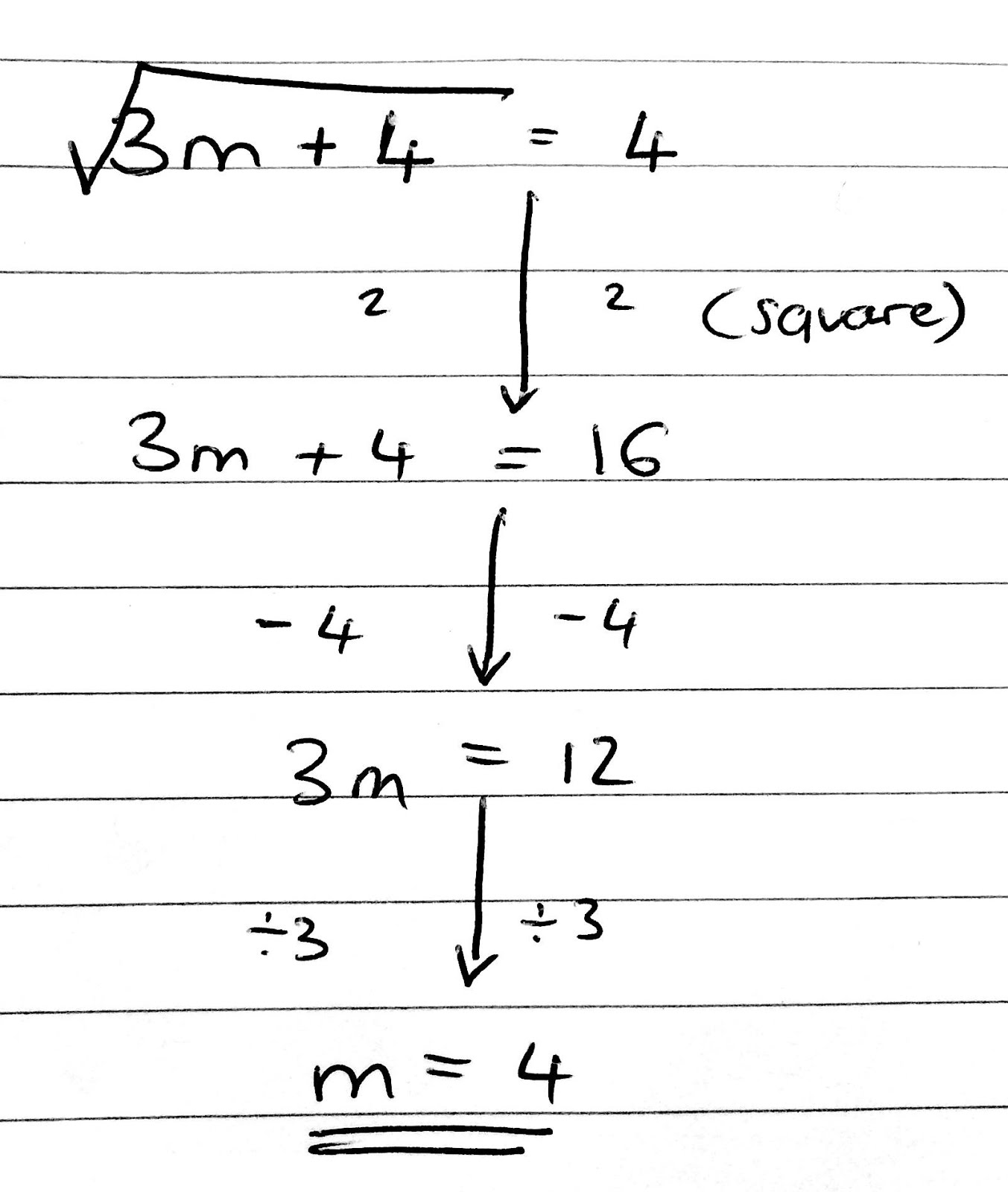I hope this two blog posts have been helpful in getting your head around algebra. Have a go at some questions? Here are three to get you started:

Find the value of x

1. x-5=12

2. 2x-2=14

3. 3(x+10)=15